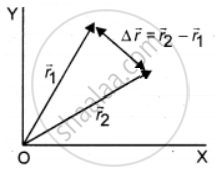Tamil Nadu Board of Secondary EducationHSC Science Class 11th

# A particle has its position moved from r→1= 3i^+4j^ to r→2= i^+2j^. Calculate the displacement vector (∆r→) and draw the r→1, r→2 and Δr→ vector in a two dimensional Cartesian coordinate system. - Physics

Sum

A particle has its position moved from vecr_1 =  3hati + 4hatj to vecr_2 =  hati + 2hatj. Calculate the displacement vector (∆vecr) and draw the vecr_1,  vecr_2 and Deltavecr vector in a two dimensional Cartesian coordinate system.

#### Solution

Given,

Position vectors vecr_1 = 3hati + 4hatj

vecr_1 = hati + 2hatj

Solution:

Displacement vector:

∆r = vecr_2 - vecr_1 = (1 - 3)hati + (2 - 4)hatj

∆r = -2hati - 2hatj = -2(hati + hatj)Concept: Distance and Displacement
Is there an error in this question or solution?

Share